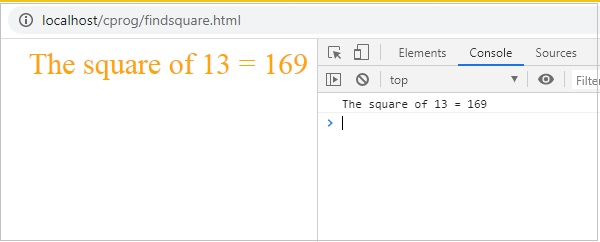# WebAssembly - Working with C

In this chapter, we are going to compile a simple C program to javascript and execute the same in the browser.

For Example − C Program

```#include<stdio.h>
int square(int n) {
return n*n;
}
```

We have done the installation of emsdk in folder wa/. In same folder, create another folder cprog/ and save above code as square.c.

We have already installed emsdk in the previous chapter. Here, we are going to make use of emsdk to compile the above c code.

Compile test.c in your command prompt as shown below −

```emcc square.c -s STANDALONE_WASM –o findsquare.wasm
```

emcc command takes care of compiling the code as well as give you the .wasm code. We have used STANDALONE_WASM option that will give only the .wasm file.

Example − findsquare.html

```<!doctype html>
<html>
<meta charset="utf-8">
<title>WebAssembly Square function</title>
<style>
div {
font-size : 30px; text-align : center; color:orange;
}
</style>
<body>
<div id="textcontent"></div>
<script>
let square; fetch("findsquare.wasm").then(bytes => bytes.arrayBuffer())
.then(mod => WebAssembly.compile(mod)) .then(module => {
return new WebAssembly.Instance(module)
})
.then(instance => {
square = instance.exports.square(13);
console.log("The square of 13 = " +square);
document.getElementById("textcontent").innerHTML = "The square of 13 = " +square;
});
</script>
</body>
</html>
```

### Output

The output is as mentioned below −